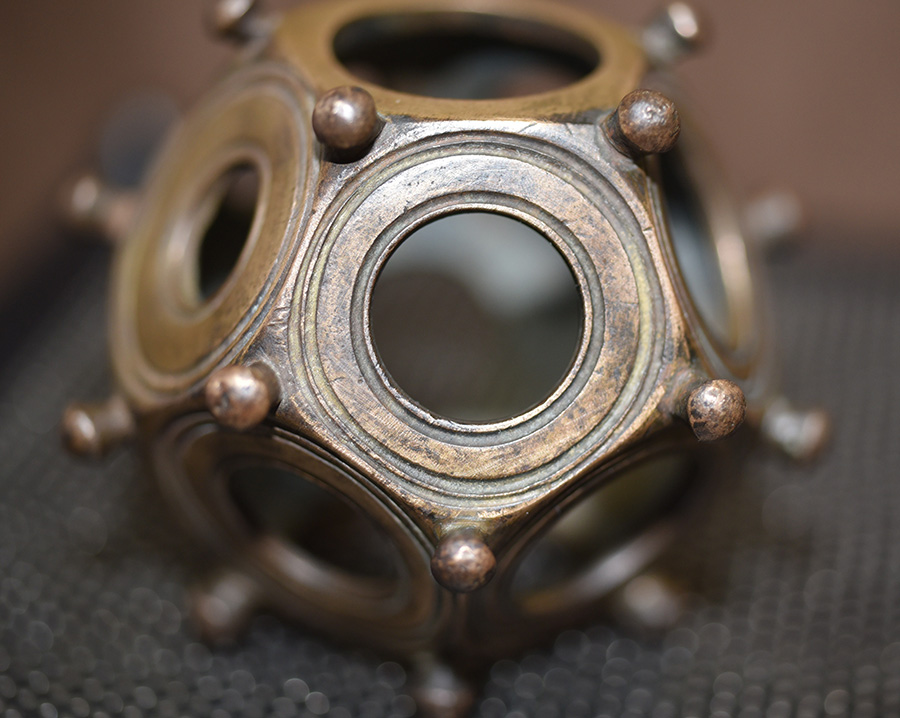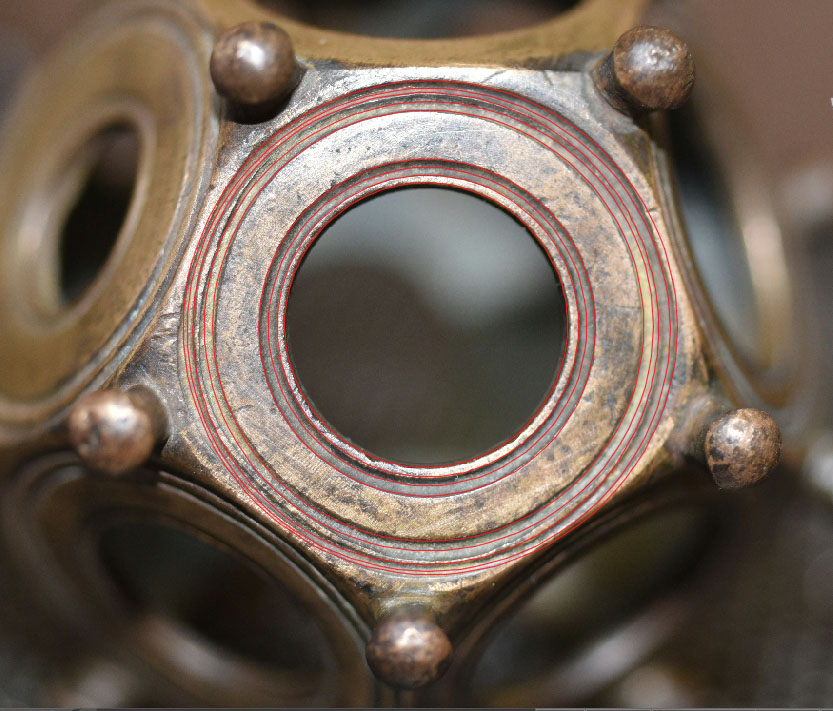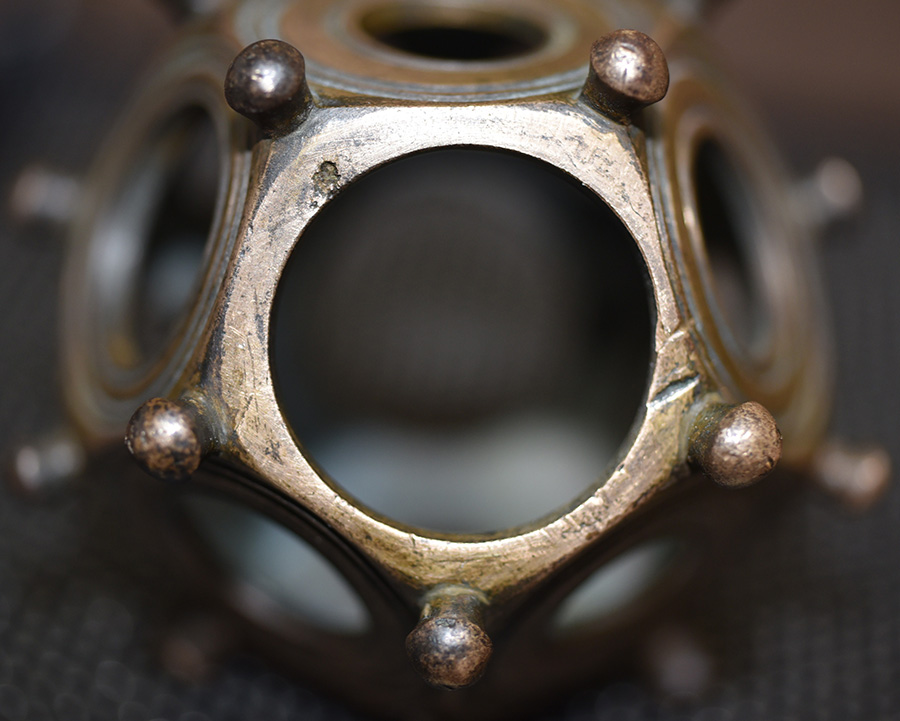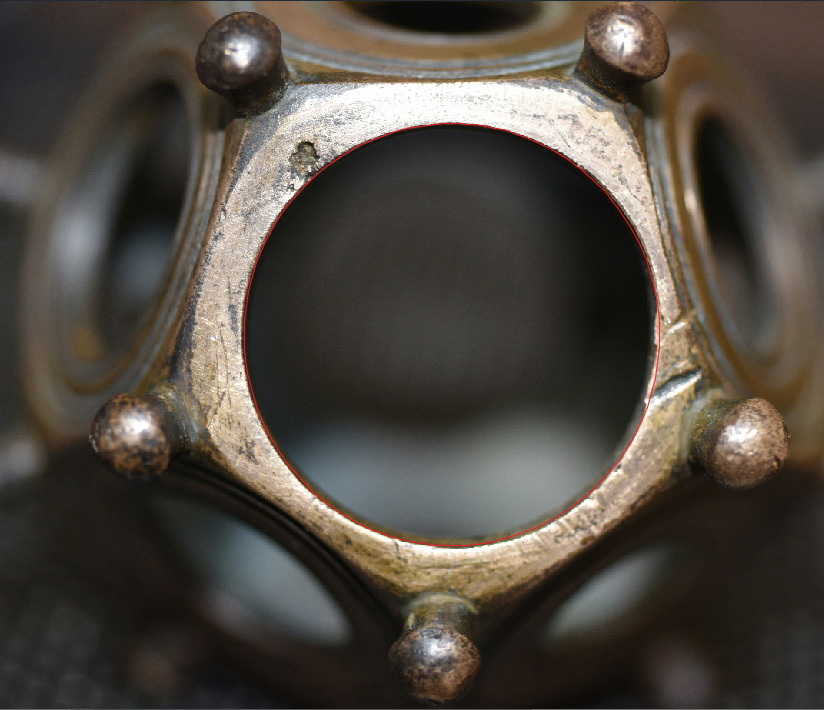FACE 6Brian Campbell's East-West measurement was 16.04 mm, which equates to .63149606". Alternatively, his North-South measurement was 16.09 mm, which equates to .63346457".

The East -West measurement was .63" to a tolerance of about 1/700th of an inch. The progression that stems from this is:

.63" and the value sets up the following mathematical progression:

.63, 1.26, 1.89, 2.52, 3.15, 3.78, 4.41, 5.04, 5.67, 6.3, 6.93, 7.56, 8.19, 8.82, 9.45, 10.08, 10.71, 11.34, 11.97, 12.6, 13.23, 13.86, 14.49, 15.12, 15.75, 16.38, 17.01, etc.

These values relate simultaneously to navigational and lunar-cycle coding. The Greek foot was 12.6" and the Greek/Hebrew reed was 126" or 10.5 English feet. The Assyrian cubit @ 25.2" was 2 Greek feet. The values 189, 378 & 756 were the quarter, half and full lengths of the Great Pyramid. The sum of 100.8' represented 1-second of equatorial arc. A "foot" measurement identified to Herodotus by Egyptian priests was 11.34" (of which here were 800 in the length of the pyramid). The sum of 1701-days would equate to 1/4th of the 6804-day lunar nutation cycle. The value 3.15 was one of the forms of PI used in certain calculations and the Sarsen Circle inner rim (based upon one of the cross-measures @ 100') is coding PI and half (so-called) Greek long stadia (630'). As mentioned, the other cross-measure was 100.8' or 1-second of arc.

The progression advances to finally generate the value 130636800' (the equatorial circumference of the Earth under the 5250' mile system).

The North-South diameter is .6336" (396/625ths) and generates the following progression:

This value was a specialised number for determining the equatorial size of the Earth, in Egyptian Royal Cubits, under 3 systems of navigation:

• The true equatorial circumference of the Earth (131383296 feet) ÷ 6336 = 20736. This figure (read as 20.736 inches) was length of the largest Egyptian Royal cubit, which mnemonically coded the 24883.2-mile (true size) assignment of 12 X 12 X 12 X 12 X 1.2 English miles of 5280' each. Under this assignment 1-minute of equatorial arc was 6082.56'.

• The "11" series (English league, mile, furlong) circumference of the Earth (130680000 feet) ÷ 6336 = 20625. This figure (read as 20.625 inches) was the length of the mid-range Egyptian Royal Cubit, which mnemonically coded the 24750-mile assignment. Under this assignment 1-minute of equatorial arc was 6050'.

• The "6 & 7" series circumference of the Earth (130636800 feet) ÷ 6336 = 20618.18182. This figure (read as 20.61818182 inches) was the length of the smallest, best known Egyptian Royal Cubit, which mnemonically coded the 24741.81818-mile assignment in English miles of 5280'. The 24741.81818 reading is also 12 X 12 X 12 X 12 X 1.2 Greek miles of 5250' or 24883.2 Greek miles. Under this assignment 1-minute of equatorial arc was 6048'.

THE FIRST OUTWARDS CONCENTRIC RING INNER EDGE

This is a perfect circle @ .672" and the mathematical progression it generates goes like this:

.672, 1.344, 2.016, 2.688, 3.36, 4.032, 4.704, 5.376, 6.048, 6.72, 7.392, 8.064, 8.736, 9.408, 10.08, etc. The progression goes on to generate all the values for 1-second of arc (100.8'), 1-minute of arc (6048') and 1-degree of arc (362880') under the Great Pyramid's literal navigational system that used the 5250' mile.

As stated, the Irish mile was 6720' or 1.28 X greater than the so-called Greek mile.

All of these measurement standards combined are clearly demonstrated in the layouts of ancient British sites like Avebury Henge, Durrington Wall's Henge, Brodgar, etc., in the distances and angles that outer marker stones or mounds sit away from the centre hubstone or obelisk.

A number like 67.2-degrees might represent the angle around from North from the hubstone. Likewise, an outer marker stone, boulder-cairn or mound might sit away from the hub position 672'. By this means ancient druids and their forebears could encode their astronomical, calendar and navigational science into the landscape in numbers. Those same numbers are encoded into mnemonic devices like the dodecahedron artefacts and were used to keep the numbers of civilisation at the forefront of memory.

The progression advances to finally generate the value 130636800' (the equatorial circumference of the Earth under the 5250' mile system).

DISTANCE TO THE CENTRE TROUGH OF THE FIRST OUTWARDS RING

This is another perfect circle @ .70875 and the progression it generates is the following:

.70875, 1.4175, 2.12625, 2.835, 3.54375, 4.2525, 4.96125, 5.67, 6.37875, 7.0875, etc. The progression is lunar and provides values for the length of the Khafre Pyramid (Egypt's pyramid of the moon ... 708.75' or 15/16ths the length of the Great Pyramid). It also generates the number for a lunar year (354.375) and the duration of the lunar nutation cycle (6804-days).

The progression advances to finally generate the value 130636800' (the equatorial circumference of the Earth under the 5250' mile system).

DISTANCE TO THE OUTER RIM OF THE 1ST CONCENTRIC RING

This is, again, a perfect circle @ .75" or 3/4ths of an inch. This progression produces the following values:

.75, 1.5, 2.25, 3, 3.75, 4.5, 5.25, 6, 6.75, 7.5, 8.25, 9, 9.75, 10.5, 11.25, 12, 12.75,13.5, 14.25, 15, 15.75, etc.The progression advances to produce a raft of numbers used in compass, lunar and navigation systems. The value goes on to generate 130636800' or 12 X 12 X 12 X 12 X 1.2 miles of 5250'.

DISTANCE TO THE INNER RIM OF THE 2ND CONCENTRIC RING.

This is very evenly set to .96" throughout the majority of the circuit, but the druidic owner has made a couple of tiny flats, undoubtedly to incorporate another code or two.

The .96" diameter generates the following progression:

.96, 1.92, 2.88, 3.84, 4.8, 5.76, 6.72, 7.68, 8.64, etc.

The North-South extremity width of Stonehenge was set out to be 384'. The distance up the sloping diagonal face of the Great Pyramid from the base to the flat top altar floor was 576'. The sum of 6720' was the Irish mile and there are 19440 Irish miles in the 24883.2 Greek mile equatorial circumference of the Earth (130636800').

The progression advances to finally generate the value 130636800' (the equatorial circumference of the Earth under the 5250' mile system).

.DISTANCE TO THE CENTRE TROUGH OF THE 2ND CONCENTRIC RING

This is best represented by 1 British standard inch throughout much of the circuit. The outer rim of the 2nd concentric ring has been fabricated with meandering walls in 4 places, where the 1" circle alternately brushes the rim side, then centres itself in the trough. The 1" diameter can therefore represent the rim edge, as well as the centre trough.

DISTANCE TO THE OUTER RIM OF THE 3RD CONCENTRIC RING

The diameter East-West is 1.0368" and the progression that stems from this goes:

1.0368, 2.0736, 3.1104, 4.1472, 5.184, 6.2208, 7.2576, 8.2944, 9.3312, 10.368, 11.4048, 12.4416, etc. The progression goes on to generate 24883.2 and ultimately 130636800' for the full equatorial circumference under the 5250' mile navigational system.

In the above lineup a value of 1036800' would be 126th of the 130636800' equatorial circumference. Also, the 130636800' equates to 24883.2-Greek miles and the sum of 1036.8 MPH would be the speed the Earth rotates around its axis in 24 hours.

The value 207360' would be 1/630th of the equatorial circumference or 207360 Greek stadia (stadiums) @ 630' each would circumnavigate the equator. Likewise, the largest Egyptian Royal cubit was 20.736". The sum of 3110.4-miles would be 1/8th of the 24883.2-mile circumference. The value 5184 is strongly represented in all manner of ancient calculations: The slope angle on the Great Pyramid is 51.84-degrees, the area that the Great Pyramid covers @ 756' per side = 72 reeds @ 10.5' each. Therefore 72 X 72 = 5184 square reeds. In the 25920-year duration of the Precession of the Equinoxes the sum of 5184-years is 1/5th of the cycle. When a leg of travel of 1 English league (16500') has been completed the circumference derived (using PI @ 1728/550ths (3.1418182) = 51840'.

The sum of 12441.6-miles = 1/2 of the 24883.2-mile circumference.

The North South diameter of this elliptical circle is 1.05' and, again, generates:

1.05, 2.1, 3.15, 4.2, 5.25, 6.3, 7.35, 8.4, 9.45, 10.5, 11.55, 12.6, 13.65, 14.7, 15.75, 16.8, 17.85, 18.9, 19.95, 21, etc. The progression goes on to generate 2551.2, 2835, 5250, 6300 etc.

This value, as 10.5' or 126" was the Hebrew Reed, as well as a reed equivalent increment used under the measurement system adopted by the Greeks, with a foot of 12.6". The value 2551.5 in days would equate to 7.2 lunar years and this value was essential to tracking the moon during 7-solar years (2556.25-days) under the lunisolar Sabbatical Calendar system, The 2835 figure represents the perimeter total, in feet, for a full circumnavigation of the Khafre Pyramid, conceived and built to be 708.75' per side or 15/16th the side length of the Great Pyramid. The 5250 figure is the Greek mile and 6300' would be 10 stadia.

DISTANCE TO THE CENTRE TROUGH OF THE 3RD CONCENTRIC RING

This is 1.0752" and codes the equatorial circumference of the Earth under the navigational system that used a mile of 5250'. The progression runs:

1.0752, 2.1504, 3.2256, 4.3008, 5.376, 6.4512, 7.5264, 8.6106, 9.6768, 10.752. The progression advances to the number 6531.84, which then doubles to 13063.6800. The equatorial size of the Earth under the Great Pyramid's coding was 130636800', and this centre trough coding was for remembering the circumference reading in feet.

DISTANCE TO THE OUTER RIM OF THE 3RD CONCENTRIC CIRCLE

This diameter is 1.1" and sets up an "11" series mathematical progression:

1.1, 2.2, 3.3, 4.4, 5.5, etc, leading on to such values as 13.2, 16.5, 17.6, 26.4, 29.7, 35.2, 52.8, 59.4, 70.4, 79.2, 82.5, 165, etc.

The sum of 1320' is a 1/4 of a mile, 1760' is 1/3rd of a mile, 2640' is 1/2 a mile, 5280' an English mile, 2970' is 1/2 an ancient Scottish mile and 5940' a full Scottish mile. The sum of 7920 English miles was the calculated diameter of the Earth and this value X 3.125 (an ancient approximation on PI for this number family) = 24750 English miles for the Equatorial circumference. Similarly, the 5280' mile X 3.125 = 1 ancient English league of 16500'. In other words, there were 7920 leagues in the equatorial circumference, or 22000 Scottish miles.

The progression advances to finally generate the value 130680000' (the equatorial circumference of the Earth under the 5280' mile system).Whereas the perfect circles and ellipse circles from the centre hole to the inner rim of the 2nd ring achieve excellent accuracy, the two rims further out meander somewhat. The diameters still comply well to codes through the majority of their circuits, sufficient to divulge the intended overall diameter to be encoded.

FACE 7Brian Campbell measured the East-West diameter of this hole to be 25 mm and the North-South diameter to be 25.5 mm.

The East-West measurement equates to .984252" and the intended diameter was, yet again, .984375", (63/64ths)as found on Face 6. The shortfall error is about 1/8000th of an inch.

The East-West code-bearing measurement is .984375" (63/64ths) and was a very important half value in ancient gold assaying. A progression stemming from this goes:

.984375, 1.96875, 2.953125, 3.9375, 4.921875, 5.90625, 6.890625, 7.875, 8.859375, 9.84375, 10.828125, 11.8125, etc. The progression goes on to produce 15.75, 29.53125 and ultimately 196.875.

The progression advances to finally generate the value 130636800' (the equatorial circumference of the Earth under the 5250' mile system).

This is essentially a lunar progression leading to 29.53125 (days) for the lunar month. However the value of 196.875-grains became the Egyptian Beqa Standard for gold weight. This standard was later adopted by both the Greeks and Romans. See Face 2 for references to the Beqa codes.

The North-South diameter @ 25.5 mm equates to 1.003937", and the intended diameter is, 1", with an overflow error of only about 1/250th of an inch.The North-South diameter is 1" and the druidic possessor of this dodecahedron could use the item as a standard for setting out measurement rules. To the left is seen two small damaged edge marks that encroach into the circle.

Continue